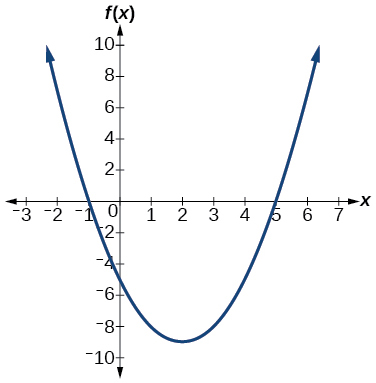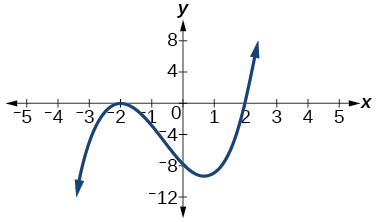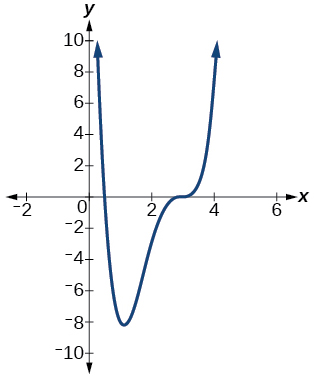# 5.8 Modeling using variation  (Page 6/14)

 Page 6 / 14

The force exerted by the wind on a plane surface varies jointly with the square of the velocity of the wind and with the area of the plane surface. If the area of the surface is 40 square feet surface and the wind velocity is 20 miles per hour, the resulting force is 15 pounds. Find the force on a surface of 65 square feet with a velocity of 30 miles per hour.

The horsepower (hp) that a shaft can safely transmit varies jointly with its speed (in revolutions per minute (rpm) and the cube of the diameter. If the shaft of a certain material 3 inches in diameter can transmit 45 hp at 100 rpm, what must the diameter be in order to transmit 60 hp at 150 rpm?

2.88 inches

The kinetic energy $\text{\hspace{0.17em}}K\text{\hspace{0.17em}}$ of a moving object varies jointly with its mass $\text{\hspace{0.17em}}m\text{\hspace{0.17em}}$ and the square of its velocity $\text{\hspace{0.17em}}v.\text{\hspace{0.17em}}$ If an object weighing 40 kilograms with a velocity of 15 meters per second has a kinetic energy of 1000 joules, find the kinetic energy if the velocity is increased to 20 meters per second.

## Chapter review exercises

For the following exercises, write the quadratic function in standard form. Then give the vertex and axes intercepts. Finally, graph the function.

$f\left(x\right)={x}^{2}-4x-5$$f\left(x\right)=-2{x}^{2}-4x$

For the following exercises, find the equation of the quadratic function using the given information.

The vertex is $\left(–2,3\right)$ and a point on the graph is $\text{\hspace{0.17em}}\left(3,6\right).$

$f\left(x\right)=\frac{3}{25}{\left(x+2\right)}^{2}+3$

The vertex is $\text{\hspace{0.17em}}\left(–3,6.5\right)\text{\hspace{0.17em}}$ and a point on the graph is $\text{\hspace{0.17em}}\left(2,6\right).$

For the following exercises, complete the task.

A rectangular plot of land is to be enclosed by fencing. One side is along a river and so needs no fence. If the total fencing available is 600 meters, find the dimensions of the plot to have maximum area.

300 meters by 150 meters, the longer side parallel to river.

An object projected from the ground at a 45 degree angle with initial velocity of 120 feet per second has height, $\text{\hspace{0.17em}}h,\text{\hspace{0.17em}}$ in terms of horizontal distance traveled, $\text{\hspace{0.17em}}x,\text{\hspace{0.17em}}$ given by $\text{\hspace{0.17em}}h\left(x\right)=\frac{-32}{{\left(120\right)}^{2}}{x}^{2}+x.\text{\hspace{0.17em}}$ Find the maximum height the object attains.

## Power Functions and Polynomial Functions

For the following exercises, determine if the function is a polynomial function and, if so, give the degree and leading coefficient.

$f\left(x\right)=4{x}^{5}-3{x}^{3}+2x-1$

Yes, degree = 5, leading coefficient = 4

$f\left(x\right)={5}^{x+1}-{x}^{2}$

$f\left(x\right)={x}^{2}\left(3-6x+{x}^{2}\right)$

Yes, degree = 4, leading coefficient = 1

For the following exercises, determine end behavior of the polynomial function.

$f\left(x\right)=2{x}^{4}+3{x}^{3}-5{x}^{2}+7$

$f\left(x\right)=4{x}^{3}-6{x}^{2}+2$

$\text{As}\text{\hspace{0.17em}}x\to -\infty ,\text{\hspace{0.17em}}f\left(x\right)\to -\infty ,\text{\hspace{0.17em}}\text{as}\text{\hspace{0.17em}}x\to \infty ,\text{\hspace{0.17em}}f\left(x\right)\to \infty$

$f\left(x\right)=2{x}^{2}\left(1+3x-{x}^{2}\right)$

## Graphs of Polynomial Functions

For the following exercises, find all zeros of the polynomial function, noting multiplicities.

$f\left(x\right)={\left(x+3\right)}^{2}\left(2x-1\right){\left(x+1\right)}^{3}$

–3 with multiplicity 2, $-\frac{1}{2}$ with multiplicity 1, –1 with multiplicity 3

$f\left(x\right)={x}^{5}+4{x}^{4}+4{x}^{3}$

$f\left(x\right)={x}^{3}-4{x}^{2}+x-4$

4 with multiplicity 1

For the following exercises, based on the given graph, determine the zeros of the function and note multiplicity.$\frac{1}{2}\text{\hspace{0.17em}}$ with multiplicity 1, 3 with multiplicity 3

Use the Intermediate Value Theorem to show that at least one zero lies between 2 and 3 for the function $\text{\hspace{0.17em}}f\left(x\right)={x}^{3}-5x+1$

#### Questions & Answers

write down the polynomial function with root 1/3,2,-3 with solution
if A and B are subspaces of V prove that (A+B)/B=A/(A-B)
write down the value of each of the following in surd form a)cos(-65°) b)sin(-180°)c)tan(225°)d)tan(135°)
Prove that (sinA/1-cosA - 1-cosA/sinA) (cosA/1-sinA - 1-sinA/cosA) = 4
what is the answer to dividing negative index
In a triangle ABC prove that. (b+c)cosA+(c+a)cosB+(a+b)cisC=a+b+c.
give me the waec 2019 questions
the polar co-ordinate of the point (-1, -1)
prove the identites sin x ( 1+ tan x )+ cos x ( 1+ cot x )= sec x + cosec x
tanh`(x-iy) =A+iB, find A and B
B=Ai-itan(hx-hiy)
Rukmini
what is the addition of 101011 with 101010
If those numbers are binary, it's 1010101. If they are base 10, it's 202021.
Jack
extra power 4 minus 5 x cube + 7 x square minus 5 x + 1 equal to zero
the gradient function of a curve is 2x+4 and the curve passes through point (1,4) find the equation of the curve
1+cos²A/cos²A=2cosec²A-1
test for convergence the series 1+x/2+2!/9x3ByByBy Gerr ZenBy RhodesBy